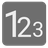Bigger Together
Elementary+
English RU UK

— But I’m so small! Why would you need me?

— Listen, even the smallest warrior can make an army so much more powerful if he’s in a right place. And even the strongest one can weaken the whole formation if he stands in the wrong rank.

Your mission here is to find a difference between the maximally positive and maximally negative numbers. Those numbers can be found by concatenating the given array of numbers.

Let’s check an example. If you have numbers 1,2,3, then 321 is the maximum number, and 123 - is the minimum. The difference between those numbers is 198. But if you have numbers 4, 3 and 12 then 4312 is the maximum number, and 1234 - is the minimum. As you can see, a simple order is not what we are looking for here.

Input: A list of positive integers.

Output: An integer.

Example:

```bigger_together([1,2,3,4]) == 3087 # 4321 - 1234
bigger_together([1,2,3,4, 11, 12]) == 32099877 # 43212111 - 11112234
bigger_together([0, 1]) == 9 # 10 - 01
bigger_together() == 0 # 100 - 100```

Precondition: All elements of the list can't be less than 0
The list can't be empty